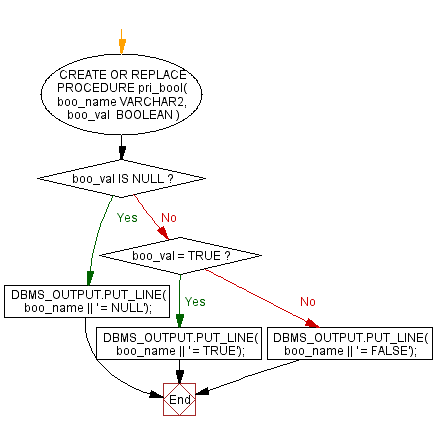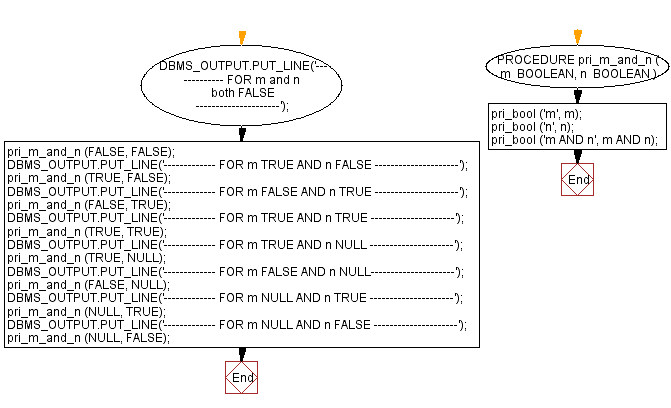﻿ PL/SQL Fundamentals Exercises: PL/SQL block to create procedure and call it for AND operator - w3resource# PL/SQL Fundamentals Exercises: PL/SQL block to create procedure and call it for AND operator

## PL/SQL Fundamentals: Exercise-12 with Solution

Write a PL/SQL block to create a procedure using the "IS [NOT] NULL Operator" and show AND operator returns TRUE if and only if both operands are TRUE.

Here is the procedure :

PL/SQL Code:

``````CREATE OR REPLACE PROCEDURE pri_bool(
boo_name   VARCHAR2,
boo_val    BOOLEAN
) IS
BEGIN
IF boo_val IS NULL THEN
DBMS_OUTPUT.PUT_LINE( boo_name || ' = NULL');
ELSIF boo_val = TRUE THEN
DBMS_OUTPUT.PUT_LINE( boo_name || ' = TRUE');
ELSE
DBMS_OUTPUT.PUT_LINE( boo_name || ' = FALSE');
END IF;
END;
/
```
```

Now call the procedure pri_bool:

PL/SQL Code:

``````DECLARE
PROCEDURE pri_m_and_n (
m  BOOLEAN,
n  BOOLEAN
) IS
BEGIN
pri_bool ('m', m);
pri_bool ('n', n);
pri_bool ('m AND n', m AND n);
END pri_m_and_n;

BEGIN
DBMS_OUTPUT.PUT_LINE('------------- FOR m and n both FALSE ---------------------');
pri_m_and_n (FALSE, FALSE);
DBMS_OUTPUT.PUT_LINE('------------- FOR m TRUE AND n FALSE ---------------------');
pri_m_and_n (TRUE, FALSE);
DBMS_OUTPUT.PUT_LINE('------------- FOR m FALSE AND n TRUE ---------------------');
pri_m_and_n (FALSE, TRUE);
DBMS_OUTPUT.PUT_LINE('------------- FOR m TRUE AND n TRUE ---------------------');
pri_m_and_n (TRUE, TRUE);
DBMS_OUTPUT.PUT_LINE('------------- FOR m TRUE AND n NULL ---------------------');
pri_m_and_n (TRUE, NULL);
DBMS_OUTPUT.PUT_LINE('------------- FOR m FALSE AND n NULL---------------------');
pri_m_and_n (FALSE, NULL);
DBMS_OUTPUT.PUT_LINE('------------- FOR m NULL AND n TRUE ---------------------');
pri_m_and_n (NULL, TRUE);
DBMS_OUTPUT.PUT_LINE('------------- FOR m NULL AND n FALSE ---------------------');
pri_m_and_n (NULL, FALSE);
END;
/
```
```

Sample Output:

```------------- FOR m and n both FALSE ---------------------
m = FALSE
n = FALSE
m AND n = FALSE
------------- FOR m TRUE AND n FALSE ---------------------
m = TRUE
n = FALSE
m AND n = FALSE
------------- FOR m FALSE AND n TRUE ---------------------
m = FALSE
n = TRUE
m AND n = FALSE
------------- FOR m TRUE AND n TRUE ---------------------
m = TRUE
n = TRUE
m AND n = TRUE
------------- FOR m TRUE AND n NULL ---------------------
m = TRUE
n = NULL
m AND n = NULL
------------- FOR m FALSE AND n NULL---------------------
m = FALSE
n = NULL
m AND n = FALSE
------------- FOR m NULL AND n TRUE ---------------------
m = NULL
n = TRUE
m AND n = NULL
------------- FOR m NULL AND n FALSE ---------------------
m = NULL
n = FALSE
m AND n = FALSE

Statement processed.

0.00 seconds
```

Flowchart:

ProcedureNow call the procedure pri_bool:Improve this sample solution and post your code through Disqus

What is the difficulty level of this exercise?

﻿